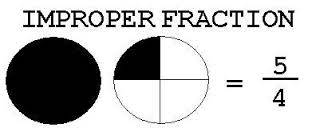What is 2.9 as an improper fraction?

2 and 9/10 of course.

thanked the writer.

The number 2.9 written as an improper fraction is twenty-nine over ten, (29/10) or twenty-nine tenths. Two and nine tenths is the mixed number or mixed fraction way of writing 2.9.To get 29/10 from 2.9 is quite straightforward, working in tenths. Obviously there are 10 tenths in whole number 1, and in 2.9, we have twenty tenths in 2, giving us 20 tenths. 0.9 as a fraction is nine-tenths or 9/10. You simply add up the number of tenths (20 + 9) to give you 29/10.

Note that 29/10 cannot be reduced to any simpler improper fraction, as 29 and 10 have no common divisors aside from 1.

In the pictured example, we can see the improper fraction 5/4 (five-quarters) is one whole set of quarters (there are 4 quarters in the number 1) with a quarter left over. Written as a mixed number, this would be one and one-quarter (1 and 1/4).

Remember, with improper fractions, the top number will always be higher than the bottom number.

For other Blurtit discussions on improper fractions, go to What Is A Improper Fraction? or What Is The Difference Between An Improper Fraction And A Mixed Number ?

If you'd like to brush up your improper fraction skills, this YouTube tutorial will come in handy:

thanked the writer.